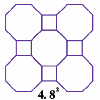#### You may also like### Schlafli Tessellations

are somewhat mundane they do pose a demanding challenge in terms of 'elegant' LOGO procedures. This problem considers the eight semi-regular tessellations which pose a demanding challenge in terms of 'elegant' LOGO procedures.### Napoleon's Theorem

Triangle ABC has equilateral triangles drawn on its edges. Points P, Q and R are the centres of the equilateral triangles. What can you prove about the triangle PQR?

# Polygon Walk

### Why do this problem?

This problem builds on ideas from the problem Vector Walk. Students are encouraged to think geometrically about vectors in order to deduce which vectors could generate particular sets of points, as well as reasoning why other sets of points could not be reached in the same way.

### Possible approach

Students could work in small groups to create some vector walks using the vector ${\bf i} = (1,0)$ and another vector ${\bf u}$. Encourage them to comment on the similarities between the points reached when different vectors ${\bf u}$ are chosen.

After students have a feel for how two vectors can be used to reach a variety of points on the plane, ask them to sketch possible arrangements of points around the origin forming an equilateral triangle, and a hexagon, using points which can be reached using the vector ${\bf i}$. Some calculation will be needed to work out the second vector needed to reach the appropriate points.

Finally, set students the last challenge to explain why a regular pentagon could not be created in the same way. Encourage them to use both algebra and geometry to justify their answer.

### Key questions

Can you draw a diagram to show an equilateral triangle or a hexagon of points around the origin?
Can you show a way to visit all these points using just ${\bf i}$ and one other vector?
What happens when you try to do the same thing with a pentagon?

### Possible extension

How might the ideas in this problem relate to finding roots of unity in the complex plane?

### Possible support

Start with the problem Vector Walk to get an idea of how we can find a set of points that can be visited according to some vector rules.